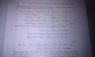# Finite Element Analysis - Author J.N Reddy Book

bugatti79
Folks,

Is there anyone out there familiar with 'An introduction to the Finite Element Method' by J.N. Reddy?

I am struggling to decipher what is happening on page 129 as shown in the attachment. If some-one is willing to help I will reply with a more specific query on that page. Thanks

#### Attachments

•IMAG0058.jpg
20.9 KB · Views: 432

Homework Helper
I think this would be easier to understand if you write it in matrix form.
Suppose you have two linear elements joined end to end, so there are 3 nodes.
If the stiffness matrices of the two elements are
$$\begin{bmatrix} k^1_{11} & k^1_{12} \\ k^1_{21} & k^1_{22} \end{bmatrix}$$
and $$\begin{bmatrix} k^2_{11} & k^2_{12} \\ k^2_{21} & k^2_{22} \end{bmatrix}$$
The assembled stiffness matrix is
$$\begin{bmatrix} k^1_{11} & k^1_{12} & 0 \\ k^1_{21} & k^1_{22}+k^2_{11} & k^2_{12} \\ 0 & k^2_{21} & k^2_{22} \end{bmatrix}$$
And similarly for the right hand side vectors.

You probably first met this idea in a dynamics course, setting up the equations of motion for mass-and-spring systems.

bugatti79
I think this would be easier to understand if you write it in matrix form.
Suppose you have two linear elements joined end to end, so there are 3 nodes.
If the stiffness matrices of the two elements are
$$\begin{bmatrix} k^1_{11} & k^1_{12} \\ k^1_{21} & k^1_{22} \end{bmatrix}$$
and $$\begin{bmatrix} k^2_{11} & k^2_{12} \\ k^2_{21} & k^2_{22} \end{bmatrix}$$
The assembled stiffness matrix is
$$\begin{bmatrix} k^1_{11} & k^1_{12} & 0 \\ k^1_{21} & k^1_{22}+k^2_{11} & k^2_{12} \\ 0 & k^2_{21} & k^2_{22} \end{bmatrix}$$
And similarly for the right hand side vectors.

You probably first met this idea in a dynamics course, setting up the equations of motion for mass-and-spring systems.

Thanks.Actually further down the page the matrix form is shown (see attached). However, I don't see how the assembled matrix you have shown for 2 linear elements can be derived 'explicitly' from the matrix attached. Ie, the second row of attached contains ##k_{11}^3## which does not exist for a system of 2 elements...? Of course we know it does not exist hence we can simply not write it in but...

#### Attachments

Homework Helper
I think the matrix in the book is slightly misleading. There should also be a vertical dotted line showing that some columns are missing, lile
$$\begin{bmatrix} \color{red}{ k^1_{11}} & \color{red}{k^1_{12}} & & \vdots \\ \color{red}{k^1_{21}} & \color{red}{k^1_{22} + k^2_{11}} & \color{blue}{k^2_{12}} & \vdots \\ & \color{blue}{k^2_{21}} & \color{blue}{k^2_{22} + k^3_{11}} & \vdots \\ \cdots & \cdots & \cdots & \ddots & \cdots & \cdots \\ & & & \vdots & k^{N-1}_{22} + k^N_{11} & \color{red}{K^N_{12}} \\ & & & \vdots & \color{red}{k^N_{21}} & \color{red}{K^N_{22}} \end{bmatrix}$$

When N = 1, you just have the first and last rows and columns forming a 2x2 matrix.

When N = 2, you have the first second and last rows and columns forming a 3x3 matrix, i.e. the matrix entries shown in red.

When N = 3, you have the entries shown in red and blue.

Last edited:
darkside00
In two elements K11^3 = 0 as there is no U4 either. Its just the notations that are generally written for more than two elements

Gold Member
As a mechanical engineer I found it much easier to lay out the matrices as AlephZero has done (i.e. graphically), the reason the author of the book lays them out in equation or linear algebra form is basically that's what you would need if you were writing your own FEA software.

Great posts AlephZero! That's some impressive application of TEX!

bugatti79
I think the matrix in the book is slightly misleading. There should also be a vertical dotted line showing that some columns are missing, lile
$$\begin{bmatrix} \color{red}{ k^1_{11}} & \color{red}{k^1_{12}} & & \vdots \\ \color{red}{k^1_{21}} & \color{red}{k^1_{22} + k^2_{11}} & \color{blue}{k^2_{12}} & \vdots \\ & \color{blue}{k^2_{21}} & \color{blue}{k^2_{22} + k^3_{11}} & \vdots \\ \cdots & \cdots & \cdots & \ddots & \cdots & \cdots \\ & & & \vdots & k^{N-1}_{22} + k^N_{11} & \color{red}{K^N_{12}} \\ & & & \vdots & \color{red}{k^N_{21}} & \color{red}{K^N_{22}} \end{bmatrix}$$

When N = 1, you just have the first and last rows and columns forming a 2x2 matrix.

When N = 2, you have the first second and last rows and columns forming a 3x3 matrix, i.e. the matrix entries shown in red.

When N = 3, you have the entries shown in red and blue.

THanks to all and particularly AlephZero. His matrix notation greatly clarifies things for me.

Appreciated.
Ed

Actually, there's a better technology than TeX for doing this. It's called "some big sheets of paper and a pack of colored pens."